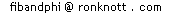The Fibonacci Puzzles page has been divided into two. Here is the SECOND part with puzzles a bit harder than those of the FIRST part which you are recommended to browse through first!

# Harder Fibonacci Puzzles

All these puzzles except one (which??) have the Fibonacci numbers as their answers.
So now you have the puzzle and the answer - so what's left? Just the explanation of why the Fibonacci numbers are the answer - that's the real puzzle!!

The Fibonacci puzzles are split into two sections: those with fairly straight-forward and simple explanations as to why the answer is the Fibonacci numbers are on the Easier Fibonacci Puzzles page.

This page contains the second set where it is not so simple to explain why their answers involve the Fibonacci numbers. Does a simple explanation exist? If you find a simple explanation please email me (click on my name at the foot of the page) and let me know as I'd like to share the simpler solutions on these pages.

0, 1, 1, 2, 3, 5, 8, 13, 21, 34, 55, 89, 144, 233, 377, 610, 987 ..More..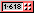## Pennies for your thoughts - Part 1

Here are two puzzles which are identical - but we count the solutions in two different ways. Each involves arranging pennies (coins) in rows.
The puzzle here is that only one of these two puzzles involves the Fibonacci number series! The other puzzle does not but just begins with a few of the Fibonacci numbers and then becomes something different. One of these puzzles is a fraud, a Fibonacci forgery. So which is the real Fibonacci puzzle?
Arrange pennies in rows under these two conditions:
1. each penny must touch the next in its row
2. each penny except ones on the bottom row touches two pennies on the row below.
There is just 1 pattern with one penny,
and 1 with two pennies
but 2 for three pennies
and 3 with four pennies as shown here:-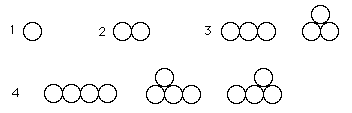The first condition means that there are no gaps in any row and the second means that upper rows are smaller than lower ones.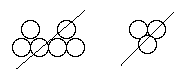The following arrangements are not proper combinations for 6 pennies
because the first has a gap in one row and the second has a penny which is not on the bottom row and is not touching two beneath it.

If there are P(n) such arrangements for n pennies,
are the P(n) numbers always Fibonacci numbers?

0, 1, 1, 2, 3, 5, 8, 13, 21, 34, 55, 89, 144, 233, 377, 610, 987 ..More..## Pennies for your thoughts - Part 2

This puzzle is the same as the previous one and again seems to involve the Fibonacci numbers - or does it?
The puzzle is exactly the same, but P(n) now counts the number of arrangements which have n pennies on the bottom row.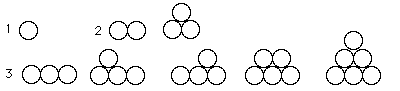Here there is only 1 arrangement with 1 penny on the bottom row so P(1)=1 and
2 arrangements with two on the bottom row, P(2)=2
and 5 patterns with a bottom row of three coins P(3)=5.
What happened to 3? F(4)=3 is missing! You can check that P(4)=13, so P(n) is clearly not the same as the Fibonacci series since F(4)=3 and F(6)=8 are missing. This time the question is
Are the P(n) numbers the alternate Fibonacci numbers:
```         i : 0 1 2 3 4 5  6  7  8
Fib(i): 1 1 2 3 5 8 13 21 34...
P(n): 1   2   5   13     ?
n : 1   2   3    4     5
```
Which one of these two Pennies puzzles is the forgery (it does not continue with a pattern of Fibonacci numbers after some point) and which one genuinely always has Fibonacci numbers of arrangements?
[With thanks to Wendy Hong for bringing these two puzzles to my attention.]

### References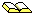Richard K Guy, The Second Strong Law of Small Numbers in The Mathematics Magazine, Vol. 63 (1990), pages 3-21, Examples 45 and 46.The first Pennies puzzle above was mentioned by F. C. Auluck in On some new types of partitions associated with Generalized Ferrers graphs in Proceedings of the Cambridge Philosophical Society, 47 (1951), pages 679-686 (examples 45 and 46).

0, 1, 1, 2, 3, 5, 8, 13, 21, 34, 55, 89, 144, 233, 377, 610, 987 ..More..## Water Treatment Plants puzzle

Cities along a river discharge cleaned-up water from sewage treatment plants. It is more efficient to have treatment plants running at maximum capacity and less-used ones switched off for a period. So each city has its own treatment plant by the river and also a pipe to its neighbouring city upstream and a pipe to the next city downstream along the riverside.
At each city's treatment plant there are three choices:
• either process any water it may receive from one neighbouring city, together with its own dirty water, discharging the cleaned-up water into the river;
• or send its own dirty water, plus any from its downstream neighbour, along to the upstream neighbouring city's treatment plant (provided that city is not already using the pipe to send it's dirty water downstream);
• or send its own dirty water, plus any from the upstream neighbour, to the downstream neighbouring city's plant, if the pipe is not being used.
 CITYA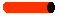CITYB~~RIVER~~

The choices above ensure that
• every city must have its water treated somewhere and
• at least one city must discharge the cleaned water into the river.

Let's represent a city discharging water into the river as "V" (a downwards flow), passing water onto its neighbours as ">" (to the next city on its right) or else "<" (to the left). When we have several cities along the river bank, we assign a symbol to each (V, < or >) and list the cities symbols in order.
For example, two cities, A and B, can

• each treat their own sewage and each discharges clean water into the river. So A's action is denoted V as is B's and we write "VV" ;
• or else city A can send its sewage along the pipe (to the right) to B for treatment and discharge, denoted ">V" ;
• or else city B can send its sewage to (the left to) A, which treats it with its own dirty water and discharges (V) the cleaned water into the river. So A discharges (V) and B passes water to the left (<), and we denote this situation as "V<".
We could not have "><" since this means A sends its water to B and B sends its own to A, so both are using the same pipe and this is not allowed. Similarly "<<" is not possible since A's "<" means it sends its water to a non-existent city on its left.

So we have just 3 possible set-ups that fit the conditions:-

 PipeFlow AB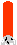RIVER ~~~~~~ Notation VV
 A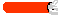B~~~~~~ >V
 AB~~~~~~ V<
Now suppose that we have more than two cities along the river bank:-
 CITYACITYBCITYC...~ ~ ~ ~ ~ ~ R I V E R ~ ~ ~ ~ ~
1. What are the eight set-ups possible for 3 cities?
2. If S(n) is the number of set-ups for n cities, then S(1)=1 and we have just shown that S(2)=3 and S(3)=8. But this does not look like the Fibonacci numbers!
What is S(4)? What is S(5)?
3. What is the relationship between the S-numbers here and the Fibonacci series!?See Fibonacci Numbers and Water Pollution Control R A Deininger in Fibonacci Quarterly, Vol 10, No 3, 1972, pages 299-300 and page 302.

0, 1, 1, 2, 3, 5, 8, 13, 21, 34, 55, 89, 144, 233, 377, 610, 987 ..More..## Wythoff's game

The Fibonacci numbers provide a winning strategy for playing a game with two piles of matches (or counters or coins etc), first described by W A Wythoff in 1906.

Players take it in turns to remove some matches (at least one) from EITHER only one pile OR ELSE an equal number from both piles. The players can decide how large each heap will be before the game starts and the winner is the one who takes the LAST match. A complete heap can be removed as your move if you like. This is not to be recommended however, since your opponent can do the same on the next move and so will win by taking the last match! This leads to the idea of "safe configurations", that is, ones from which it is possible to force a win, no matter what your opponent does.

For further details, see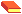T. H. O'Beirne Puzzles and Paradoxes, Dover press, 1965, chapter 8.Ball, W.W.R. and Coxeter, H.S.M. Mathematical Recreations and Essays, 13th edition, Dover Publications, 1987. A great classic with plenty to keep you amused and enthused on Maths - definitely worth buying! (You can order it online via the title-link.)

0, 1, 1, 2, 3, 5, 8, 13, 21, 34, 55, 89, 144, 233, 377, 610, 987 ..More..### Non-neighbour Groups

How often have the list of names in your class been read out in alphabetical order, or you have been asked to line up in alphabetical order for a fire-practice or when the results of a test are given out? The trouble with this is that you are always next to the same one or two people that are on either side of you in the alphabetical order - your alphabetical neighbours. You will have got to know them quite well over the course of a year, so this puzzle is about meeting other people who are not your alphabetical neighbours.
Suppose that part of the class is needed for a particular task or game. Let's also say that the group should contain no alphabetical neighbours in it, so it gives everyone in the group a chance to team up with new people.
In how many ways can you choose such a group from a class of N students?

For instance, if there are 3 people in the class, let's label them according to their position when in the alphabetical order, so they are 1, 2 and 3.

The puzzle is to select a group from the class
with no pair of successive numbers (positions) in the group.
So if 1 is in the group, then 2 cannot be and 3 may be or not; so we have the groups:
{1} and {1,3}

If 2 is in the group then, since both 1 and 3 are 2's alphabetical neighbours, then that group will consist of 2 alone!
{2}
If 3 is in the group then 2 cannot be and 1 may be. But remember that the group with 3 and 1 in it has already been included above! So we have the following possible new groups with 3 in:
{3}
All the possible groups of non-neighbours are:
{1,3}     {1}    {2}     {3}     {}
Did you notice that the group {} with nobody in it is a non-neighbour group too? So from a class of 3 people, there are 5 ways to pick a group consisting solely of non-neighbours. How many are there in a class of size 4? or 5? or 6? Why?The Fibonacci Split D L Silverman, J Rec Maths, 9 (1976/7), page 298 is the origin of the mathematics behind this puzzle.On The Number of Fibonacci Partitions of a Set Helmut Prodinger Fibonacci Quarterly Vol 19, 1981, pages 463 - 465 generalises this problem.

## Another one!

On the previous puzzle page, Aphrodite was playing with her coloured rods but Rodrigo had taken all those of length 1 in the No one! puzzle. This time she has her unit rods back and another set of length 1 rods of a new colour too, so she has two colours of rods of length 1.
In how many ways can she make a line of length N if there are two kinds of length 1 rods (of different colours)
Remember that all rods of the same length have the same colour and different lengths are different colours, with two colours of unit length rods.
In this puzzle we are interested in the colours along the lines of length N.
 length 1length 2length 3length 4length 5... ...
• There are now 2 "lines" of colours which are of length 1:• and 5 ways to make a line of length 2:• Can you find all 13 of length 3?
• How many are there of length 4?
• and how many of length 5?
• Can you guess how many there will be of length N? Rest your mouse here for a hint
• Can you find a general method of generating all solutions of length N using the solutions of smaller length?Problem H-641 Fibonacci Numbers count compositions, solution by Emeric Deutsch Fibonacci Quarterly Vol 45, 2007, pages 286-7

## That's odd!

Aphrodite, Rodrigo and Rodney, who love to play with coloured rods, are now joined by a new friend, Rodderick.
The rods are of different lengths (whole numbers), all the rods of the same length having the same colour and there are lots of each colour and all the sizes you could want.
In the 2 puzzles of the first Puzzle page:
• in No One! there were no rods of length 1
• in Ones and Twos the only rods available were of lengths 1 or 2
• the Another one!, the previous puzzle on this page, we had two different colours of rod of length 1
This time Rodderick has all the rods of odd lengths: 1, 3, 5, 7, etc.
The puzzle this time is to help Rodderick count the number of different coloured patterns (arrangements) of rods in a line for a given total length N.
• there are 3 ways to make a line of length 4:
4 is 1111, 13 and 31
The last two, 13 and 31 are different since the order of the colours is different.
• Find the 5 ways to make a line of length 5 using only the odd-length rods
• How many ways are there using odd rods to make a line of length 6?
• and of length 7?
• Guess the number of solutions for a line of length N
• Find a method of generating the solutions of length N if you have already found all solutions of smaller lengths

## Steven and Todd

This puzzle is about collections of integers with a restriction on the maximum size.
Steven and Todd are playing a game. They each take turns to name a number bigger than the previous one, Steven always choosing an even number (2,4,6,...) and Todd only an odd (1,3,5,...). Either of them can end the game at any time but, to prevent it going on for ever, they agree on a maximum number for each game.
How many different number games are there for a given maximum when Todd starts?
E.g. if the maximum is 3, we have the following lists:
1. 1
2. 1 2
3. 3
4. 1 2 3
Don't forget the game when Todd starts but ends it straight away: the list of numbers played is empty!
So there are 5 lists when Todd starts and they do not play beyond 3.
If they didn't go beyond 2, then only the top two are allowed, together with the empty list making 3 lists.
What happens if they increase the maximum number to 4? All the above lists still apply, but you can add some more that will include 4. How many extra lists will there be?
Extend the lists some more by adding those with 5 and so on, noting how many there are for each choice of maximum.

Now try the same puzzle but with Steven always starting:

How many different number games are there for a given maximum when Steven starts?

Finally put all the results together:

How many different number games are there for a given maximum when either of them can start?Two challenging problems in Combinatorics section 17 of Mathematical Chestnuts from Around The World, R Honsberger, Mathematical Association of America (2001) where this problem is called Terquem's Problem after Olry Terquem who solved it in 1839.

0, 1, 1, 2, 3, 5, 8, 13, 21, 34, 55, 89, 144, 233, 377, 610, 987 ..More..### Series and Parallel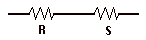If we have two electrical resistances of R ohms and S ohms in series: then the combined resistance is just R+S ohms.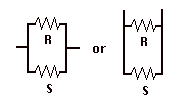You'll remember that if we have 2 resistances R and S in parallel: then the combined resistance, T, is given by

```                  1   1   1
- = - + -
T   R   S
```

### Rungs

Suppose we extend the pattern of parallel resistors into longer and longer ladders, by putting a 1 ohm resistor between two wires and then keep adding single ohm resistors in parallel. What is the total resistance?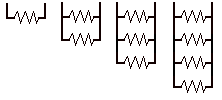...
In the diagram above, the 2 resistor ladder has two 1-ohm resistors in parallel so their combined resistance R is given by the equation:
```    1/R   = 1/1   + 1/1 = 2         so    R=1/2
```

For the 3 resistor ladder, we have combined the 2 resistor ladder with another resistor of 1-ohm, in parallel, so the combined resistance S here is

```     1/S = 1/(1/2) + 1/1 = 2+1 = 3   so   S=1/3
```
Try computing the overall resistance for yourself for 4 resistors, then with 5 and 6.

What pattern are you getting for the combined resistance?

Can you prove that your pattern always holds?

### Rungs and One Side

Now try it with the following pattern of resistances, where one of the legs of the ladder also has resistance of 1-ohm and we alternately add a resistor on a side leg and then on a rung: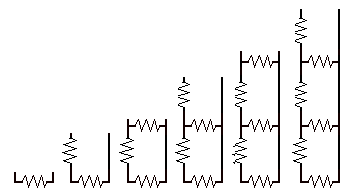The first ladder has a single resistor so is 1 ohm.
The second ladder has two resistors in series, so the combined resistance is 2.
The third ladder has a 1 ohm resistor in parallel with the second ladder (2 ohms), so the combined resistance S of 1 ohm and 2 ohms in parallel is
` 1/S = 1/1 + 1/2 = 3/2   i.e. S=2/3`
Similarly, the next ladder has a 1 ohm resistor in series with the previous ladder, so its total resistance is 1+2/3=5/3.

What about the next two ladders? What is the general pattern now?

Again, can you prove that your pattern will always hold?

### One Side only

Try making a ladder where the only resistances are DOWN ONE SIDE and there is no resistance on the "rungs". What pattern do you get now?

### Have you the Capacity for another puzzle?

Replace the resistors with capacitors in the Rungs and One Side puzzle.
What pattern do you get now?
[Suggested by Bhushit Joshipura.]

### References on the Resistance LaddersThe Golden Ratio in an Electrical Network, J Wlodarski in The Fibonacci Quarterly Vol 9 (1971) pages 188 and pg 194.Generalization of Modified Morgan-Voyce Polynomials, Fibonacci Quarterly Vol 38 No 1, 2000, pages 8-16.
An advanced mathematical article dealing with resistors, capacitors and inductors.The appearance of Fibonacci Numbers and the Q Matrix in electrical network theory S L Basin Mathematics Magazine (1963) pages 84-97

0, 1, 1, 2, 3, 5, 8, 13, 21, 34, 55, 89, 144, 233, 377, 610, 987 ..More..## A Fibonacci Jigsaw puzzle or How to Prove 64=65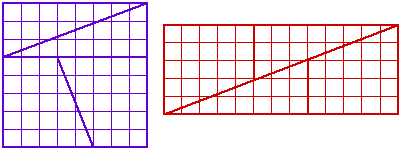The 8-by-8 blue square in the diagram here can be cut up into 4 pieces that, when rearranged, make the red 5-by-13 rectangle. But the blue square contains 8x8=64 little squares whereas the red rectangle contains 5x13=65. Where has the extra square come from?

This puzzle can be repeated with other consecutive Fibonacci numbers,

`replace 5, 8 and 13  by  8, 13 and 21  or by 3, 5 and 8`
If you look at the "8, 13, 21" jigsaw, the square is 13x13=169 but this time the rectangle is 8x21=168 so we have lost a square this time! Sometimes there is a square extra, sometimes a square goes missing.

### Not convinced?

Try this demonstration

Try cutting out the pieces as shown and rearranging them yourself if you are not sure the puzzle "works".
It works even better as a class demonstration using an overhead projector: photocopy the square with its grid lines onto an overhead projector transparency, cut out the shapes and show them as a square on the screen, then rearrange it into the rectangle, carefully lining up the grid lines to "show I'm not cheating"!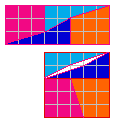or look at this diagram of the pieces using a 3-by-8 rectangle and a 5-by-5 square where the 5-by-5 square is 1 unit larger than the 3-by-8 rectangle.

### But what is the explanation?

Hints:
1. What is the formula behind these puzzles?
Why do some of the square arrangements gain a small unit square and others lose one?
For any three consecutive Fibonacci numbers: F(n-1), F(n) and F(n+1), it relates F(n)2 to F(n-1)F(n+1); what is it?
Perhaps you can try to prove it is always true.
2. Now look carefully at one of the jigsaw puzzles. Is it really what it seems? Try taking a different angle on the problem - perhaps looking at it from a tangent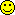.

Some References on this puzzle:Edward Wakeling in Rediscovered Lewis Carroll Puzzles Dover, 1995, says that this puzzle was found in Lewis Carroll's papers (the original is now kept at Princeton University) and that this puzzle goes back to Schlomilch, 1868.Martin Gardner's Mathematics, Magic and Mystery a 1956 Dover book, is a book with magic tricks and how the mathematics behind them makes them work. It has two chapters on such Geometrical Vanishes and has a full explanation of this and other puzzles. He also traces its origins back to Sam Loyd (senior) who presented it to the American Chess congress (using an 8-by-8 chessboard) in 1858, ten years before Wakeling's reference to Schlomilch in the reference above. However this also appears not to be the earliest reference...David Wells in The Penguin Book of Curious and Interesting Puzzles (Penguin, 1997) in Puzzle 143 traces its origin back to William Hooper in Rational Recreations of 1774.

## How to Prove 64=63!!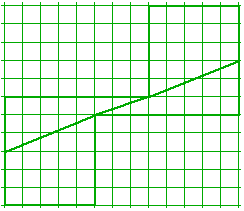The blue jigsaw of area 64 little squares, when re-arranged into the red positions with 65 little squares, had seemingly gained a square.

Here is another arrangement. This time the blue puzzle's pieces have been re-arranged as shown here in green and now it loses a square -- there are two 5-by-6 rectangles + 3 squares in a row joining them, making a total area of 63!

So what's happened this time???Fibonacci Sequences and a Geometrical Paradox A F Horadam Mathematics Magazine 35 (1962) pages 1-11, gives an extensive analysis of the problem and shows that with the difference of 1 unit of area, the puzzle extends to each triple of Fibonacci numbers.Ball, W.W.R. and Coxeter, H.S.M. Mathematical Recreations and Essays, 13th edition, Dover Publications, 1987 has only the first version of the puzzle (page 85) and says the earliest reference the authors can find is Zeitschrift für Mathematik und Physik Leipzig, 1868, vol XIII, page 162.On Fibonacci Sequences and a Geometrical Paradox Santosh Kumar Mathematics Magazine 37 (1964), pages 221-223 analyses both versions.The second version comes from Henry E Dudeney's 536 Puzzles and Curious Problems (reprinted 1983); Problems 352 and 353 and their answersMartin Gardner's Mathematics, Magic and Mystery a 1956 Dover book (mentioned in the first version of this puzzle) says that Sam Loyd junior (who adopted his father's name and continued his father's puzzle columns) was the first to discover this new reduced-square version. This book has a good explanation of how the two puzzles work and that the Fibonacci numbers produce other sizes of puzzle with identical variations of an additional and missing single square. He shows how other generalized Fibonacci sequences (i.e. starting with two other numbers rather than 0 and 1) can be used to devise variations where any number of squares can be made to appear and disappear, together with many other kinds of geometrical dissection puzzles. If you like the puzzles on these two Web pages, you'll enjoy this book too with number, handkerchief and card puzzles based on mathematics.

## Yet another Fibonacci Jigsaw Puzzle!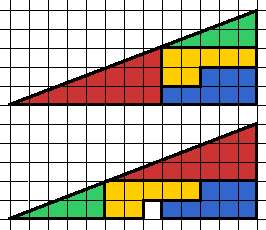Roy Nauw of Kloetinge, the Netherlands found another Fibonacci Puzzle. His lecturer, Floor van Lamoen, mentioned it on the Geometry Puzzles newsgroup (archived at Math Forum) and it is copied here with Roy's permission (and my thanks to them both).

It is made up of 4 pieces,

• a smaller green triangle with height 2 and base 5 ;
• a larger red triangle with height 3 and base 8;
• an orange L-shape of height 2 and width 5;
• a blue L-shape of the same width and height but a different shape.
The two L-shaped pieces fit together to make a 3-by-5 rectangle. They can all be arranged into a 13-by-5 triangle as shown here. Rearranging the 4 pieces shows the triangle has a square missing!
Where does the hole come from?

What's the answer this time and how is it connected with the Fibonacci Numbers?

The puzzle will work with a green triangle height 1 base 3 and a red triangle height 2 base 5, and two straight pieces (1-by-3) that make up a 2-by-3 rectangle. Rearranging them this time makes the small rectangle 1 square smaller this time so the two straight pieces have to overlap.
Similarly, using triangles of height 3 base 8 and height 5 base 13 the rectangle again loses one square.

small green
triangle
large red
triangle
rectangle
green width
red height
height x base = Area
rectangle
red width
green height
height x base = Area
Rectangle Area
Difference
heightbase heightbase
smaller
puzzle
13 25 2 x 3 = 6 1 x 5 = 5-1
puzzle
above
25 38 3 x 5 = 15 2 x 8 = 16+1
larger
puzzle
38 513 5 x 8 = 40 3 x 13 = 39-1
larger
puzzle
513 821 8 x 13 = 104 5 x 21 = 105+1

0, 1, 1, 2, 3, 5, 8, 13, 21, 34, 55, 89, 144, 233, 377, 610, 987 ..More..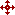The Amazing Mathematical Object Factory has an interesting section on Fibonacci Numbers which contains explanations for some of the puzzles on this page and the relationships between them.© 1996-2021 Dr Ron Knott